There are three main ways to check the correctness of derivative specifications:

• Specify the FD= or FDHESSIAN= option in the PROC NLP statement to compute finite-difference approximations of first- and second-order derivatives. In many applications, the finite-difference approximations are computed with high precision and do not differ too much from the derivatives that are computed by specified formulas.

• Specify the GRADCHECK option in the PROC NLP statement to compute and display a test vector and a test matrix of the gradient values at the starting point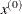by the method of Wolfe (1982). If you do not specify the GRADCHECK option, a fast derivative test identical to the GRADCHECK=FAST specification is done by default.

• If the default analytical derivative compiler is used or if derivatives are specified using the GRADIENT or JACOBIAN statement, the gradient or Jacobian computed at the initial pointis tested by default using finite-difference approximations. In some examples, the relative test can show significant differences between the two forms of derivatives, resulting in a warning message indicating that the specified derivatives could be wrong, even if they are correct. This happens especially in cases where the magnitude of the gradient at the starting pointis small.

The algorithm of Wolfe (1982) is used to check whether the gradient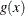specified by a GRADIENT statement (or indirectly by a JACOBIAN statement) is appropriate for the objective function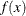specified by the program statements.

Using function and gradient evaluations in the neighborhood of the starting point, second derivatives are approximated by finite-difference formulas. Forward differences of gradient values are used to approximate the Hessian element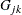,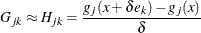where
is a small step length and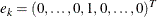is the unit vector along theth coordinate axis. The test vector, with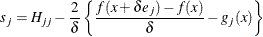contains the differences between two sets of finite-difference approximations for the diagonal elements of the Hessian matrix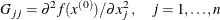The test matrix
contains the absolute differences of symmetric elements in the approximate Hessian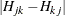,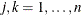, generated by forward differences of the gradient elements.

If the specification of the first derivatives is correct, the elements of the test vector and test matrix should be relatively small. The location of large elements in the test matrix points to erroneous coordinates in the gradient specification. For very large optimization problems, this algorithm can be too expensive in terms of computer time and memory.